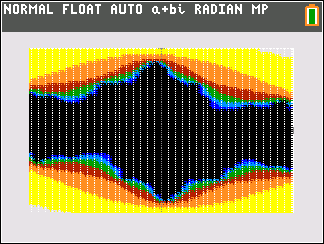## Monday, May 27, 2019

### Fractal Plots: TI-84 Plus CE and HP Prime

Fractal Plots:  TI-84  Plus CE and HP Prime

Introduction

The program CHAOS1 plots a fractal to simulate a Julia Set.  Given the complex numbers z_k and c, repeats the equation:

z_n = z_n-1 + c^2

The program will ask for a sample graphing space with the a border B.  The viewing window will be set up as:

Xmin = -B
Xmax = B
Ymin = -B
Ymax = B

The program CHAOS1 will also as for the number of points (G), this will determine the number of points plotted in the graph.  The higher G is, the more detailed the fractal is; however, the plot will take longer to complete.

We start with each point on the graph z_0 = a + b*i, where i = √-1.  Then we calculate:

z_1 = z_0^2 + c
z_2 = z_1^2 + c
z_3 = z_2^2 + c
and so on.

For each z_0, we have two possibilities for the repeated calculations:

1.  |z_n| = abs(z_n) eventually goes towards infinity or

2.  |z_n| = abs(z_n) eventually settles (or converges) to a specific point.

A color is assigned to each point z_0.  The color is determined by the amount of iterations it takes to reach |z| > 2.  For such points that fit criteria 2, and never reaches |z| >2, the point is colored black.  This method creates the Julia set for any given c.

For the program CHAOS1, I set up a rank of colors to plot each point, and arbitrary pick a maximum amount of iterations.  For example, I picked 9 colors for the TI-84 Plus CE version.  Hence for each point, if |z| ≤ 2 after 9 iterations, color the point black.

Obviously the more levels (colors) we have, the more accurate our fractal is.  With the programs listed, you can adjust the number of colors.   Keep in mind the TI-84 Plus CE has up to 15 colors, the Casio fx-CG50 has up to 7, and the HP Prime's colors have 256 levels of red, green, and blue.

You can download CHAOS1 for both the TI-84 Plus CE and HP Prime here:
https://drive.google.com/open?id=1A06e_pAPk7fMik07Pbi1tyjbLSUkVUN2

TI-84 Plus CE Program CHAOS1  (File:  CHAOS1.8xp)

For the Ti-84 Plus CE, I recommend the number of grid points of no more than 75.

The list of colors that I use in this code is:

1.  WHITE:  color value of 20
2. LTGRAY: color value of 21
3.  YELLOW: color value of 19
4.  ORANGE:  color value of 15
5.  BROWN: color value of 16
6.  GREEN: color value of 14
7.  LTBLUE:   color value of 18
8.  BLUE:  color value of 10
9.  BLACK: color value of 12

Note:  ⅈ represents √-1 ([Shift] [ 3 ])

Func: a+b ⅈ
FnOff: ClrDraw
Input "BORDER: ",B
Input "NO. GRID PTS: ",G
Input "PT C X+Yi: ", C
{20,21,19,15,16,14,18,10,12} → L6
dim(L6) → S
-B → Xmin: B → Xmax
-B → Ymin: B → Ymax
For(I, -B, B, 2B/G)
For(J, -B, B, 2B/G)
1 → K
I + J ⅈ→ Z
Lbl A
If abs(Z)>2 or K=S
Goto B
Z^2 + C → Z
1 + K → K
Goto A
Lbl B
L6(K) → L
Pt-On(I, J, 3, L)
End

ExamplesB = 1, G = 75, C = -0.79 + 0.15iB = 1, G = 75, C = 0.334 - 0.169i

HP Prime Program CHAOS1 (File:  CHAOS1.hpprgm)

I set 18 color levels, starting from white to black.   Because of the faster processor and calculating speed, I have increased the number of grid points to 500.  250 makes a picture with lines.

Note:  I use the parenthesis notation (x,y) for the complex number x + yi because the imaginary character i unfortunately does not transfer to computer text.  (updated 5/27/2019 - this allows for copying the code to the HP Prime connectivity kit/emulator)

EXPORT CHAOS1()
BEGIN
// 2019-05-19 EWS
LOCAL b,g,c,l6,s;
LOCAL i,k,j,z,l;
STARTAPP("Function");
INPUT( {b, g, {c, [,] }, "Fractal",
{ "Border: ", "Grid: ", "C: "},
{ "Min/Max - X/Y", "# Grid Pts", "C: x + yi " });
// size the screen
Xmin:=-b; Xmax:=b;
Ymin:=-b; Ymax:=b;
// clear the screen
RECT();
// list of colors
l6:= { #FFFFFFh, #C0C0C0h,
#D0D0D0h, #FFFF00h,
#B0B0B0h, #FF8000h,
#905000h, #400808h,
#00FF00h, #004060h,
#003000h, #00FFFFh,
#80D0FFh, #0000FFh,
#000080h, #400080h,
#404040h, #000000h};
// plotting
s:=SIZE(l6);

FOR i FROM -b TO b STEP (2*b)/g DO
FOR j FROM -b TO b STEP (2*b)/g DO
k:=0;

REPEAT
k:=k+1;
IF k==1 THEN
z:=(i,j);
ELSE
z: = z^2 + c;
END;
UNTIL ABS(z)>2 OR k==s;

l:=l6(k);
PIXON(i,j,l);
END;
END;

WAIT(0);
END;

ExamplesB = 1, G = 500, C = 0.38 + 0.008iB = 1, G = 500, C = -0.11 -0.8i

Have fun!  Explore the possibilities,

Eddie

All original content copyright, © 2011-2019.  Edward Shore.   Unauthorized use and/or unauthorized distribution for commercial purposes without express and written permission from the author is strictly prohibited.  This blog entry may be distributed for noncommercial purposes, provided that full credit is given to the author.

### Swiss Micros DM16L: Advanced Boolean and Factorial (up to 20)

Swiss Micros DM16L:   Advanced Boolean and Factorial (up to 20) Introduction The program listing, for the Swiss Micros DM16L and Hewlett Pac...